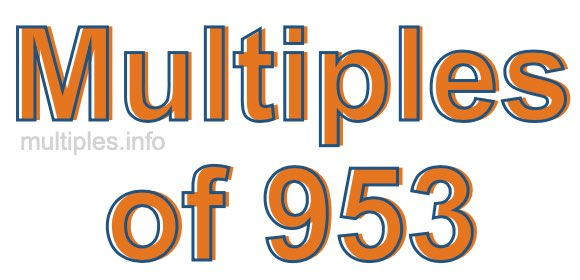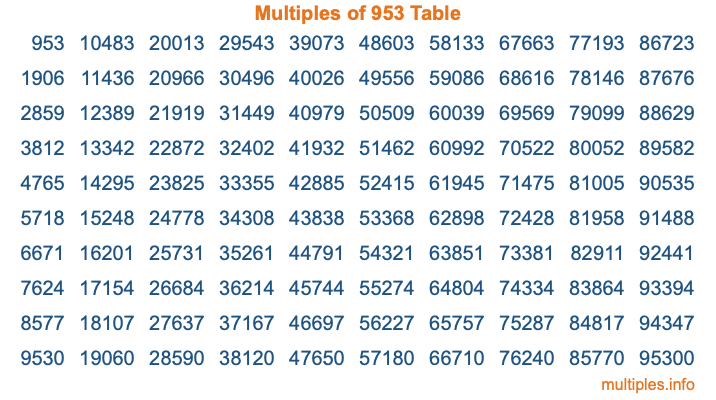Multiples of 953Welcome to the Multiples of 953 page. Here we will first teach you everything you will ever need to know about the multiples of 953, and then give you a study guide summary of everything we taught you to make sure you remember it all. Use this page to look up facts and learn information about the multiples of 953. This page will make you a multiples of nine hundred fifty-three expert!

Definition of Multiples of 953
Multiples of 953 are all the numbers that when divided by 953 equal an integer. Each of the multiples of 953 are called a multiple. A multiple of 953 is created by multiplying 953 by an integer.

Therefore, to create a list of multiples of 953, you start with 1 multiplied by 953, then 2 multiplied by 953, then 3 multiplied by 953, and so on for as long as you want. Thus, the list of the first five multiples of 953 is 953, 1906, 2859, 3812, and 4765. To see a larger list of multiples of 953, see the printable image of Multiples of 953 further down on this page. We also have a category where you can choose any nth multiple of 953.

Multiples of 953 Checker
The Multiples of 953 Checker below checks to see if any number of your choice is a multiple of 953. In other words, it checks to see if there is any number (integer) that when multiplied by 953 will equal your number. To do that, we divide your number by 953. If the the quotient is an integer, then your number is a multiple of 953.

Is  a multiple of 953?

Least Common Multiple of 953 and ...
A Least Common Multiple (LCM) is the lowest multiple that two or more numbers have in common. This is also called the smallest common multiple or lowest common multiple and is useful to know when you are adding our subtracting fractions. Enter one or more numbers below (953 is already entered) to find the LCM.

Check out our LCM Calculator if you need more details about the Least Common Multiple or if you need the LCM for different numbers for adding and subtraction fractions.

nth Multiple of 953
As we stated above, 953 is the first multiple of 953, 1906 is the second multiple of 953, 2859 is the third multiple of 953, and so on. Enter a number below to find the nth multiple of 953.

th multiple of 953

Multiples of 953 vs Factors of 953
953 is a multiple of 953 and a factor of 953, but that is where the similarities end. All postive multiples of 953 are 953 or greater than 953. All positive factors of 953 are 953 or less than 953.

Below is the beginning list of multiples of 953 and the factors of 953 so you can compare:

Multiples of 953: 953, 1906, 2859, 3812, 4765, etc.

Factors of 953: 1, 953

As you can see, the multiples of 953 are all the numbers that you can divide by 953 to get a whole number. The factors of 953, on the other hand, are all the whole numbers that you can multiply by another whole number to get 953.

It's also interesting to note that if a number (x) is a factor of 953, then 953 will also be a multiple of that number (x).

Multiples of 953 vs Divisors of 953
The divisors of 953 are all the integers that 953 can be divided by evenly. Below is a list of the divisors of 953.

Divisors of 953: 1, 953

The interesting thing to note here is that if you take any multiple of 953 and divide it by a divisor of 953, you will see that the quotient is an integer.

Multiples of 953 Table
Below is an image of the first 100 multiples of 953 in a table. The table is in chronological order, column by column. The first column has the first ten multiples of 953, the second column has the next ten multiples of 953, and so on.The Multiples of 953 Table is also referred to as the 953 Times Table or Times Table of 953. You are welcome to print out our table for your studies.

Negative Multiples of 953
Although not often discussed or needed in math, it is worth mentioning that you can make a list of negative multiples of 953 by multiplying 953 by -1, then by -2, then by -3, and so on, to get the following list of negative multiples of 953:

-953, -1906, -2859, -3812, -4765, etc.

Multiples of 953 Summary
Below is a summary of important Multiples of 953 facts that we have discussed on this page. To retain the knowledge on this page, we recommend that you read through the summary and explain to yourself or a study partner why they hold true.

There are an infinite number of multiples of 953.

A multiple of 953 divided by 953 will equal a whole number.

953 divided by a factor of 953 equals a divisor of 953.

The nth multiple of 953 is n times 953.

The largest factor of 953 is equal to the first positive multiple of 953.

953 is a multiple of every factor of 953.

953 is a multiple of 953.

A multiple of 953 divided by a divisor of 953 equals an integer.

953 divided by a divisor of 953 equals a factor of 953.

Any integer times 953 will equal a multiple of 953.

Multiples of a Number
Here you can get the multiples of another number, all with the same attention to detail as we did for multiples of 953 on this page.

Multiples of
Multiples of 954
Did you find our page about multiples of nine hundred fifty-three educational? Do you want more knowledge? Check out the multiples of the next number on our list!